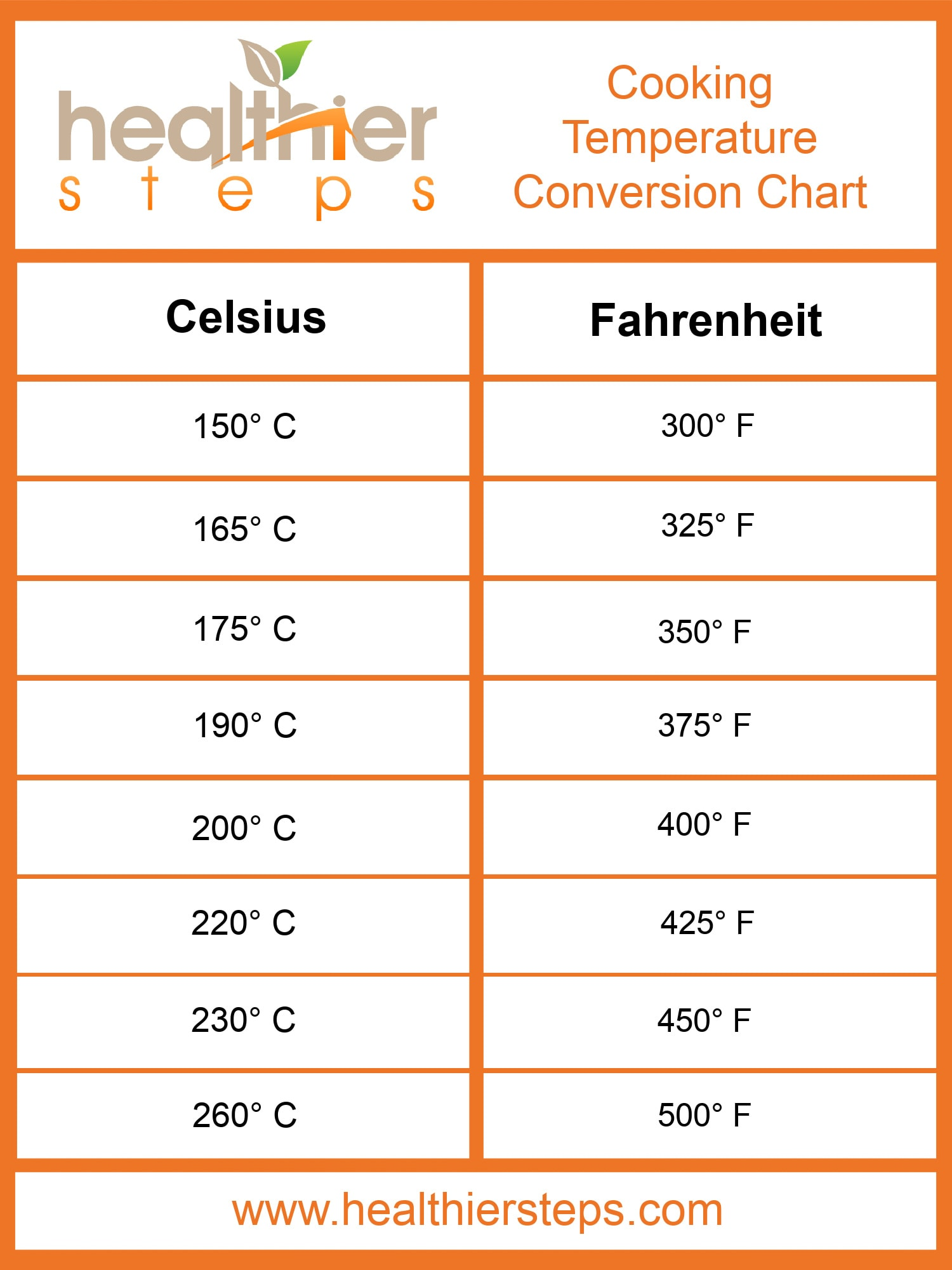# Oven Temperature Conversion – Fahrenheit & Celsius

When it comes to cooking, knowing how to convert oven temperatures between Fahrenheit and Celsius can be incredibly useful. Whether you’re following a recipe from a different country or using an older recipe with different temperature settings, being able to convert between these units of measurement will ensure that your dishes turn out just right.

There are two main temperature scales used in cooking: Fahrenheit and Celsius. In the United States, Fahrenheit is the most commonly used scale, while Celsius is widely used in many other parts of the world. To convert between the two, you can use a simple formula: Celsius = (Fahrenheit – 32) / 1.8 or Fahrenheit = (Celsius * 1.8) + 32.

Here’s a handy conversion table to help you easily convert between Fahrenheit and Celsius:

Fahrenheit Celsius
275°F 135°C
300°F 150°C
325°F 165°C
350°F 175°C
375°F 190°C
400°F 205°C

Now you can confidently convert oven temperatures between Fahrenheit and Celsius, ensuring that your cooking adventures are a success!

## The Basics of Oven Temperature Conversion

The Fahrenheit and Celsius scales are two common temperature measurements used in cooking. Fahrenheit is commonly used in the United States, while Celsius is more commonly used in many other parts of the world. Converting between the two scales is relatively simple once you understand the formulas.

Here are the basic formulas for converting between Fahrenheit and Celsius:

Fahrenheit to Celsius: C = (F – 32) x 5/9 F = (C x 9/5) + 32

For example, if a recipe calls for an oven temperature of 350°F and you want to convert it to Celsius, you can use the Fahrenheit to Celsius formula:

C = (350 – 32) x 5/9 = 176.67°C

Similarly, if a recipe calls for an oven temperature of 180°C and you want to convert it to Fahrenheit, you can use the Celsius to Fahrenheit formula:

F = (180 x 9/5) + 32 = 356°F

By understanding these basic conversion formulas, you can easily convert oven temperatures between Fahrenheit and Celsius, ensuring your dishes are cooked to the right temperature.

## Converting Fahrenheit to CelsiusTo convert Fahrenheit to Celsius, one can use the formula: Celsius = (Fahrenheit – 32) * 5/9. It involves subtracting 32 from the Fahrenheit value and then multiplying the result by 5/9.

Here is a conversion table that provides some common Fahrenheit to Celsius conversions:

Fahrenheit Celsius
32°F 0°C
50°F 10°C
68°F 20°C
86°F 30°C
104°F 40°C

Converting Fahrenheit to Celsius is a straightforward process that can be done using a simple formula. Understanding this conversion allows for better comprehension and comparison of temperature values between the Fahrenheit and Celsius scales.

## Converting Celsius to FahrenheitConverting temperatures from Celsius to Fahrenheit can be useful when working with different temperature scales. By knowing how to convert between these two scales, you can easily understand temperature measurements in either scale.

To convert Celsius to Fahrenheit, you can use the following formula:

Fahrenheit = (Celsius × 9/5) + 32

This formula states that to convert Celsius to Fahrenheit, you need to multiply the Celsius temperature by 9/5 and then add 32 to the result. This will give you the equivalent temperature in Fahrenheit.

For example:

Celsius Fahrenheit
0 °C 32 °F
25 °C 77 °F
100 °C 212 °F

Using this formula and the given conversion table, you can easily convert any Celsius temperature to Fahrenheit. It’s a simple calculation that can be done manually or with the help of a calculator.

## Tips and Tricks for Successful Oven Temperature ConversionConverting oven temperatures from Fahrenheit to Celsius (or vice versa) can be a tricky task, but with a few tips and tricks, you can successfully convert and cook your dishes to perfection.

1. Use a Conversion Chart: One of the easiest ways to convert oven temperatures is by using a conversion chart. These charts provide a quick reference for converting between Fahrenheit and Celsius temperatures, saving you time and effort. You can easily find online conversion charts or create one of your own to keep in your kitchen for easy reference.

• Example Conversion Chart:
Fahrenheit Celsius
275°F 135°C
300°F 150°C
325°F 165°C
350°F 180°C
375°F 190°C

2. Adjust Cooking Time: When converting oven temperatures, it’s important to remember that cooking times may need to be adjusted. Different ovens have slight temperature variations, so to ensure your dish is cooked perfectly, you may need to adjust the cooking time slightly. Keep an eye on your dish as it cooks and use a thermometer to check for doneness.

3. Double-Check the Recipe: Before starting any recipe, it’s crucial to double-check the oven temperature mentioned. Sometimes recipes may have incorrect temperature conversions, leading to undercooked or overcooked dishes. Always cross-reference the given temperature with a reliable source or conversion chart to ensure accuracy.

By following these tips and tricks, you can easily convert oven temperatures and cook your dishes at the desired temperature, resulting in delicious meals every time.